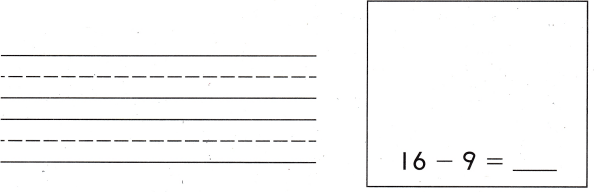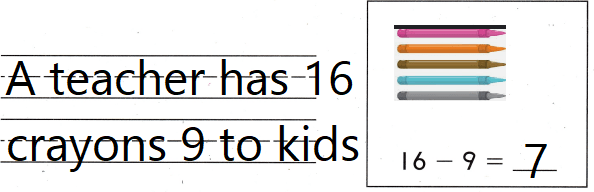# Texas Go Math Grade 1 Lesson 8.3 Answer Key Generate Subtraction Word Problems

Refer to our Texas Go Math Grade 1 Answer Key Pdf to score good marks in the exams. Test yourself by practicing the problems from Texas Go Math Grade 1 Lesson 8.3 Answer Key Generate Subtraction Word Problems.

## Texas Go Math Grade 1 Lesson 8.3 Answer Key Generate Subtraction Word Problems

Essential Question
How can a subtraction sentence help you write a subtraction story problem?
Explanation:
6 – 5 = 1
This is the example of a subtraction sentence
Story:
Mica has 6 apples and ate 5. how many are left?
6 – 5 = 1

Explore
9 – 3 = ___
Draw to show a story problem. Then solve.

9 – 3 = ___
Explanation:
9 – 3 = 6
Explanation:
Fruits in the basket are 9. 3 are strawberries the rest are plums. How many plums are in the basket?
9 – 3 = 6
so, 6 are plums.

For The Teacher

• Have children tell a story problem using the subtraction fact, Then have them use two-color counters to solve the problem. Have them draw simple pictures to represent the story problem.
Explanation:
Fisherman catches 5 fishes in the net.5 fishes are sold. How many are in the net now.
5 – 5 = 0
This is a subtraction factBy using the color counters done the fraction facts.

Math Talk
Mathematical Processes
Tell your story problem to a classmate. How are your story problems alike?
Explanation:
He used the subtraction fact as 6 -6 = 0

Model and Draw

Write a story problem for the fact 5 – 3 = ___. Draw a picture to solve.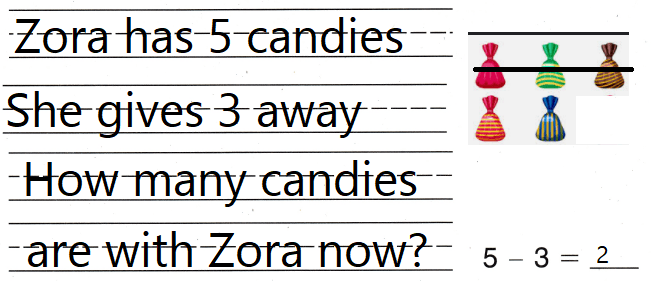Explanation:
Written a story problem for subtraction sentence.

Share and Show

Write a story problem for the subtraction fact. Draw a picture to solve.

Question 1.
12 – 5 = ___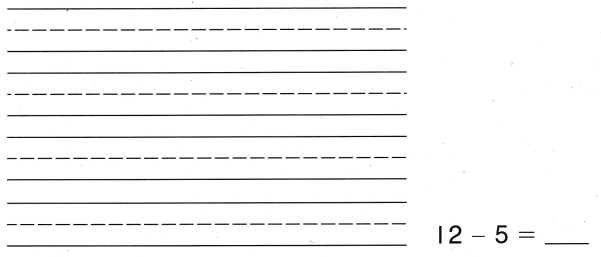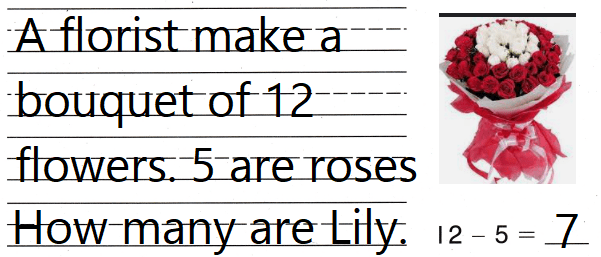Explanation:
Written a story problem for subtraction sentence.

Problem Solving

Write a story problem for the subtraction fact. Draw a picture to solve.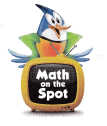Question 2.
H.O.T. 10 – __ = 6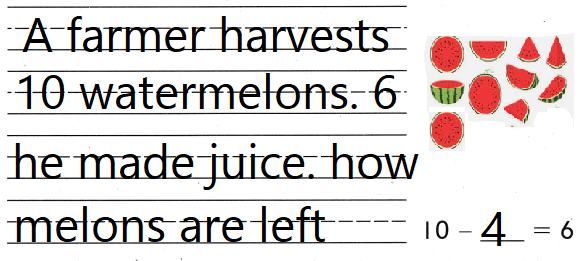Explanation:
Written a story problem for subtraction sentence.

Question 3.
H.O.T. Multi-step ___ – ___ = 4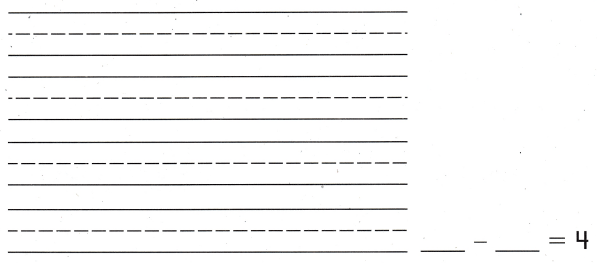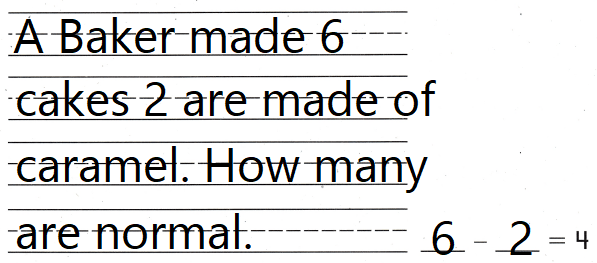Explanation:
Written a story problem for subtraction sentence.

Question 4.
Which subtraction fact matches the drawing?(A) 10 – 6 = 4
(B) 6 – 5 = 1
(C) 11 – 6 = 5
Explanation:
11 – 6 = 5 matches the subtraction fact matches the drawing.

Question 5.
Which drawing matches the subtraction fact?
3 – 2 = ___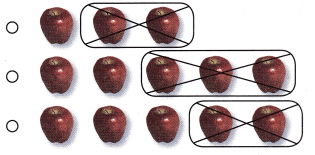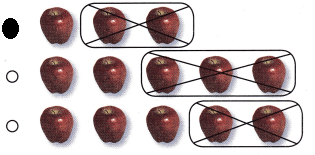Explanation:
Bubbled the correct drawing.

Question 6.
Use Tools Cara made this story problem for 9 – 7 = ___. 9 hens are in a barn. How many hens are in the barn now? Which sentence did Cara forget to write in the story problem?
(A) 7 hens join them.
(B) 7 hens fly out.
(C) 7 cows are in the barn.
Explanation:
9 – 7 =2. 9 hens are in a barn.
7 hens fly out. 2 hens are in the barn.

Question 7.
Texas Test Prep Which subtraction sentence matches the model?(A) 9 – 6 = 3
(B) 6 – 3 = 3
(C) 9 – 3 = 6
Explanation:
6 -3 = 3 is the subtraction sentence matches the model

Take Home Activity

• Have your child draw o picture to solve 11 – 3. Then have him or her write a story problem for 11 – 3.

### Texas Go Math Grade 1 Lesson 8.3 Homework and Practice Answer Key

Write a story problem for the subtraction fact. Draw a picture to solve.

Question 1.
16 – 7 = ___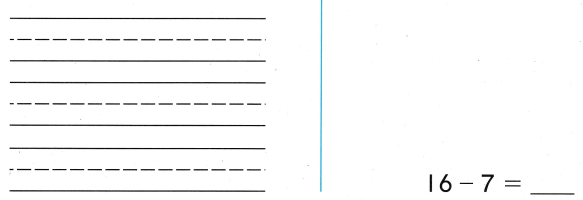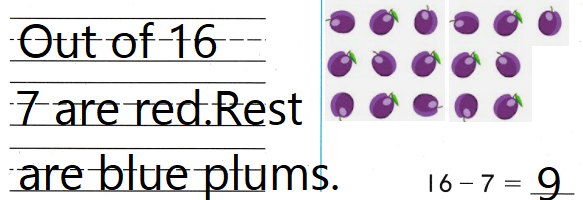Explanation:
Written a story problem for subtraction sentence.

Problem Solving

Write a story problem for the subtraction fact. Draw a picture to solve.

Question 2.
___ – ___ = 8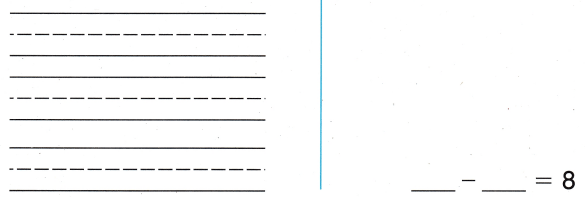Explanation:
Written a story problem for subtraction sentence.

Texas Test Prep

Lesson Check

Question 3.
Which subtraction fact matches the drawing?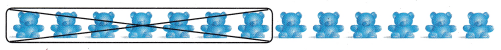(A) 12 – 7 = 5
(B) 13 – 7 = 6
(C) 7 – 6 = 1
Explanation:
13 – 7 = 6
Matches the drawing

Question 4.
Which drawing matches the subtraction fact?
5 – 2 = ___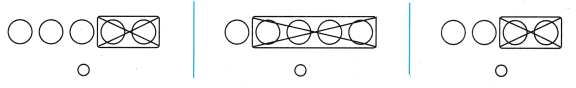Explanation:
Bubbled the correct match
5 – 3 = 2

Question 5.
Which subtraction fact matches the cubes?(A) 6 – 3 = 3
(B) 9 – 3 = 6
(C) 9 – 6 = 3